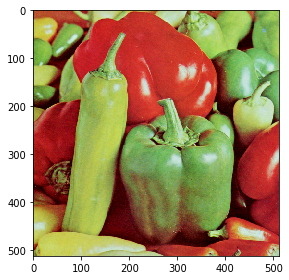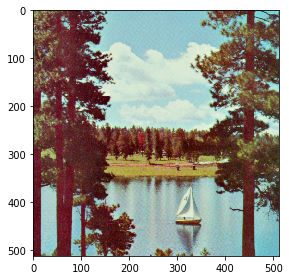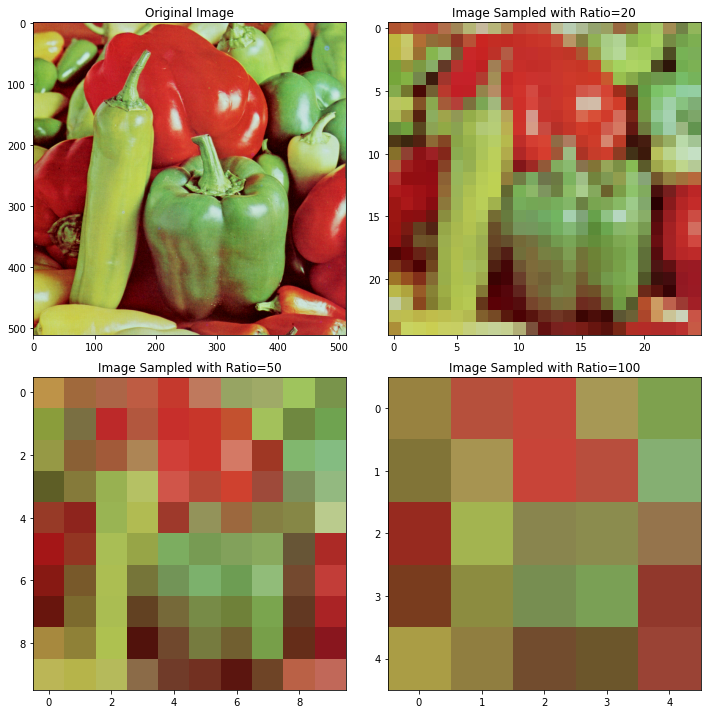Betul Mescioglu

# Sampling and Quantization of Images

## Sampling:

### Description:

Sampling is the process of converting a continuous-space signal into a discrete-space signal. In image processing, we have to convert analog images into digital ones to display them on electronic devices. This process also makes it easier and more flexible to perform operations on images as we can decrease the number of pixels as we please. An image may be continuous on x- and y- coordinates. To convert it to digital form, we have to apply sampling in both coordinates. If we have a colored image, we have to perform sampling on all channels as well.

### Algorithm:

First we select a sampling ratio which also determines the resolution of the resulting image. The higher the ratio, the less resolution the image will have. This sampling ratio becomes the height and width of a square that we trace along the image. We start from top left and take the average value of the pixels covered by this square and register this value as the resulting image's first pixel value. Then move the square to the right by sampling ratio, take the average value of the pixels covered by the square again. This is done until we reach the end of the row. Then we move to square down by the sampling ratio and do the same process for this row as well. We repeat this until we reach the bottom of the image. This process is done for all three channels if we are dealing with a colored image. The resulting image's height and width can be found by dividing the height and the width of the original image by the sampling ratio.

``````#import necessary libraries
import numpy as np
import matplotlib.pyplot as plt
import skimage.io``````
``````#Loading original image , 
skimage.io.imshow(peppers)
plt.show()
skimage.io.imshow(boat)
```````<matplotlib.image.AxesImage at 0x247996f33a0>```````print(peppers.shape)
print(type(peppers))
print(boat.shape)
print(type(boat))``````
```(512, 512, 3)
<class 'numpy.ndarray'>
(512, 512, 3)
<class 'numpy.ndarray'>
```

### Sampling function:

This function creates an all-zero-array, then it goes through the image by sampling ratio increments and calculates the average values of the encompassed pixels and saves them to the created array. Finally returns this array as the sampled image.

``````def sample(image, sampling_ratio):

image_sampled = np.zeros((int(image.shape/sampling_ratio), int(image.shape/sampling_ratio), image.shape), dtype='float32')
for i in range(image_sampled.shape):
for j in range(image_sampled.shape):
for k in range(image_sampled.shape):
sample = image[i*sampling_ratio:(i+1)*sampling_ratio, j*sampling_ratio:(j+1)*sampling_ratio, k]
image_sampled[i,j,k] = np.mean(sample)

return image_sampled.astype('uint8')``````

### Results with Different Sampling Ratios:

``````#Drawing the original and the sampled images with ratios of 20, 50 and 100
plt.figure()
fig, ax = plt.subplots(ncols=2,nrows=2, figsize=(10,10))
ax = ax.ravel()
fig.tight_layout()
#Plot the original image
ax.imshow(peppers)
ax.set_title('Original Image')
#Plot the image sampled with ratio 20
ax.imshow(sample(peppers, 20))
ax.set_title('Image Sampled with Ratio=20')
#Plot the image sampled with ratio 50
ax.imshow(sample(peppers, 50))
ax.set_title('Image Sampled with Ratio=50')
#Plot the image sampled with ratio 100
ax.imshow(sample(peppers, 100))
ax.set_title('Image Sampled with Ratio=100')``````
`Text(0.5, 1.0, 'Image Sampled with Ratio=100')`
`<Figure size 432x288 with 0 Axes>```````#Drawing the original and the sampled images with ratios of 20, 50 and 100
plt.figure()
fig, ax = plt.subplots(ncols=2,nrows=2, figsize=(10,10))
ax = ax.ravel()
fig.tight_layout()
#Plot the original image
ax.imshow(boat)
ax.set_title('Original Image')
#Plot the image sampled with ratio 20
ax.imshow(sample(boat, 20))
ax.set_title('Image Sampled with Ratio=20')
#Plot the image sampled with ratio 50
ax.imshow(sample(boat, 50))
ax.set_title('Image Sampled with Ratio=50')
#Plot the image sampled with ratio 100
ax.imshow(sample(boat, 100))
ax.set_title('Image Sampled with Ratio=100')``````
`Text(0.5, 1.0, 'Image Sampled with Ratio=100')`
`<Figure size 432x288 with 0 Axes>`